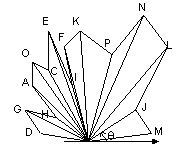```

Simple closed path: through every points, does not intersect itselfMethod: order points according to Żc to an anchor point

How to find Żc=tan-1 (y/x)?

Żc= tan-1 (y/x)            Ī¶ in (I) ČHŁŁ

<=> y/x                       Ī¶ in (I) ČHŁŁ<=> 0 Īž y / (x + y) Īž 1     Ī¶ in (I) ČHŁŁ

i.e.,Program

t := y / |x| + |y|;   (0, x = y = 0)

if x < 0 then 	        (II, III)

t := 2 ĪV t;

else if y < 0 then    (IV)

t := 4 ĪV t;

0 Īž t Īž 4

0 Īž 90t Īž 360

```